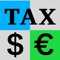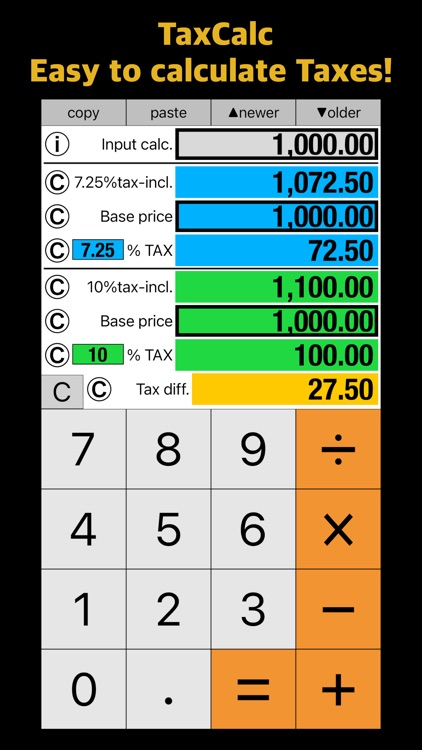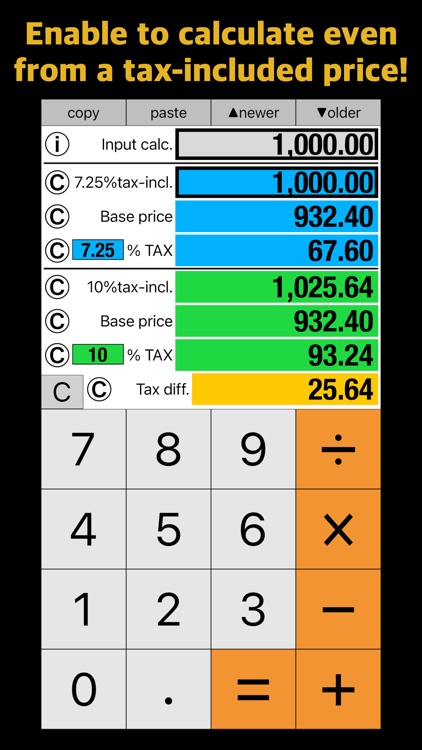## "Tax Calculator - TaxCalc” is a calculator that calculates Tax amount# TAX calculator - TaxCalc

by Takao Ichimura"Tax Calculator - TaxCalc” is a calculator that calculates Tax amount. It calculates Tax amount and others by using a calculated input price as a tax-included price or a Base price.### App Details

Version
1.0.0
Rating
NA
Size
12Mb
Genre
Utilities Productivity
Last updated
August 28, 2021
Release date
August 28, 2021

### App Store Description

"Tax Calculator - TaxCalc” is a calculator that calculates Tax amount. It calculates Tax amount and others by using a calculated input price as a tax-included price or a Base price.

The result price columns (tax-incl., Base price, TAX) are divided into upper and lower with different tax rates, and the two tax rates are calculated together. Each tax rate can be changed by tapping the tax rate column.

After calculation, a frame will be added around the Input calc. column, and the Input calc. price will be entered in the result price column with a frame, and the tax amount and others will be calculated.

You can add a frame around the Base price column or the tax-incl. column by tapping it.

If there are frames around the both upper and lower base columns, Input calc. price will be in the both base columns, and the tax amount and others will be calculated.

If there is a frame around the tax-incl. column, Input calc. price will be in the tax-incl. column, and the tax amount and others will be calculated. If the Input calc. price is not suitable to the tax-included price (e.g. Input calc. price is 2 and 7.25% tax-incl.), corrected Input calc. price will be in the tax-incl. column with red color. The upper and the lower are calculated using the same Base price.

Tax diff. is the value by subtracting the upper TAX amount from the lower TAX amount.

After calculation, if you tap the Base price column or the tax-incl. column without a frame, the frame will move to the column and other prices will be recalculated.

If you don’t need an input calculation, tap “=“ key after entering a price in Input calc. column.

Instead of tapping "=" key, you can tap a result column.

Tap “copy” key to copy Input calc. price into clipboard.
Tap "Ⓒ" button to copy a price of each column into clipboard.

In the tax calculation of this calculator, the number of digits after the decimal point is 2 and the 3rd digit after the decimal point is rounded off.

The developer assumes no responsibility for any loss or claims by third parties which may arise from the use of this app.

"Tax Calculator - TaxCalc" is one of the Dentaku series apps.
Dentaku series has the following variations.
• Dentaku: General basic calculator
• Modulo Calculator: Quotient and remainder of division
• TaxCalc: TAX calculator
• DentakuTax: Japanese consumption tax calculator
• MediCal: Calculation of required number of sheets and tablets in dispensing
• IndiaCalc: Indian numbering system adapted